Home  │ Audio Home Page

Created September 29, 2010.  See Document History at end for details.

# A Linear Logarithmic Volume Control

### Introduction

Human hearing spans a volume range of about a million to one.  In order to hear the range from the quietest whisper to the loudest gun shot, sounds are scaled so that differences in volume are heard in ratios rather than linearly.  Every 2 to 1 difference in sound level registers about the same change regardless of absolute level.  Thus it is said that our hearing scale is logarithmic.   A linear adjustment used alone would only allow about 20dB of useful adjustment in its through most of its range, the rest of the lower level crowded at the bottom of the adjustment as shown in figure 1.  For this reason audio volume controls are created with an uneven resistance pattern meant to match logarithmic hearing as much as possible.  This graded resistance taper however would be expected to compare unfavorably with a linear potentiometer with its even distribution of resistance due to the difficulty and imprecision of modulation a precisely changing resistance pattern.  If somehow a circuit could create a logarithmic gain change with a linear potentiometer, greater volume accuracy and tracking between channels could be expected.  Now to present one circuit concept that accomplishes just this.
 Figure 1:  Logarithmic Plot of a Linear Control (x-axis is %adjustment; y-axis is dB gain)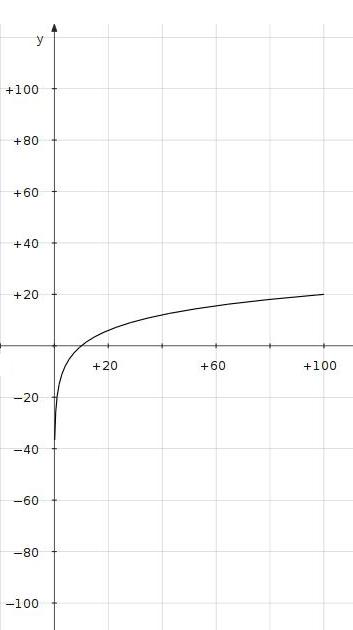### An Illustrative Circuit

Figure 2:  Control Circuit 1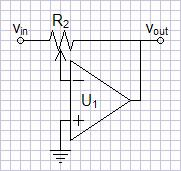A circuit putting the resistance of one end of the potentiometer in the numerator and the other in the denominator of the gain equation would seemingly expand the range of logarithmic gain change considerably.  The circuit of figure 2 does just that.  The gain equation relative to control adjustment is:
 AV = - R2a R2b = x R2-x where x represents control adjustment from 0 - R2 on feedback side of the wiper.
In normalized form the equation becomes:
 AV = - x 1-x where x represents control adjustment from 0 - 1 on feedback side of the wiper.
The plot of figure 3 to the right shows a logarithmic range of approximately 40dB.  At the extremes of adjustment the gain goes to the extremes of zero and infinity (In reality, limited to the open loop gain of the operational amplifier.).  A perfect logarithmic adjustment would never go to zero gain (-infinity dB).  As a result even a log-taper potentiometer has a limited logarithmic range to allow the bottom adjustment to drop to zero.  This circuit is best to illustrate the concept but is unfeasable due to possibility of infinite gain.

 Figure 3:  Logarithmic Plot of Control Circuit 1 (x-axis is %adjustment; y-axis is dB gain)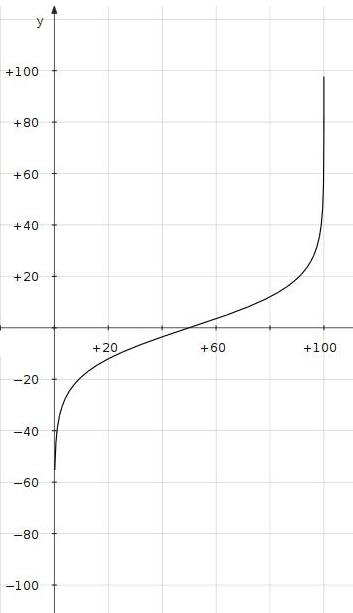### A More Practical Circuit

Figure 4:  Control Circuit 2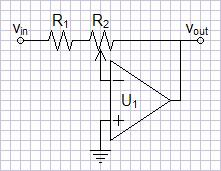The circuit of figure 2 above would be improved by preventing infinite gain.  The circuit of figure 4 adds a fixed resistor R1 before the potentiometer to this end.  This resistor also prevents the possiblity of the zero input impedance that would result at the high extreme of adjustment.  The plot of figure 5 now shows the gain limited at the upper end of the logarithmic range.  The maximum gain will be:
 AV,MAX = - R2 R1

 Figure 5:  Logarithmic Plot of Control Circuit 2 (x-axis is %adjustment; y-axis is dB gain)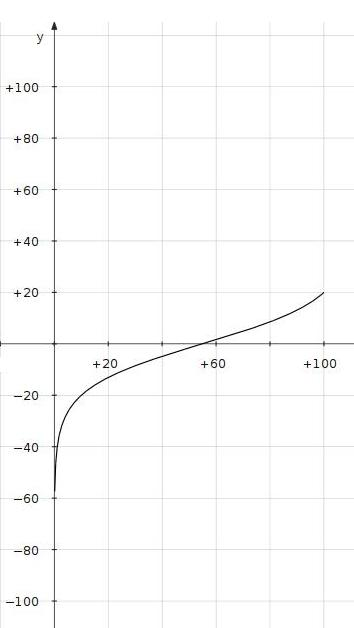### Spice Simulation

I generally expect simple op-amp circuits to function correctly as their feedback circuits dictate.  In spite of believing that an op-amp stable for a gain of one in a non-inverting configuration could operate down to a gain of zero in the inverting configuration, I needed to verify my assumption.  Modeling an LT1007 op-amp on LTSpice IV, I could take the gain down almost to zero with no problems.  At zero gain a harmless anomaly cropped up:
 Figure 6:  Zero Gain AC SPICE Analysis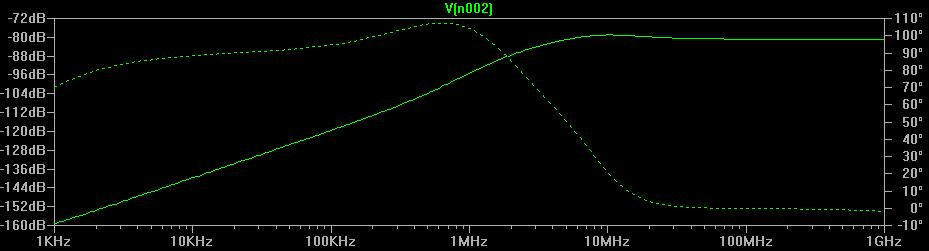Basically, this is a rise in the ultrasonic frequency response from zero that raises the 20kHz response to -133dB.  I expect this is a voltage divider effect between R1+R2 and a complex op-amp output impedance.  This is because output impedance of the op-amp will rise above the dominant pole in a way consistent with these results.  Although this is of no real consequence, you can eliminate it by adding a small resistance into the feedback loop between vout and R2.  Adding a value of 10Ω to values of R1=1.1kΩ and R2=10kΩ resulted in a fix with a limitation that gain could only be turned down to -60dB.

### Final Circuit Correcting a Potential Operational Error

Figure 7:  Control Circuit 3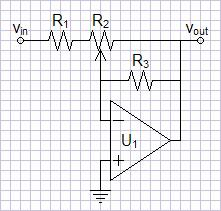A concern that might not be as obvious has to be addressed.  It is possible that the potentiometer wiper could lose contact momentarily.  This could be a undetectably brief open during normal operation or worse if the control becomes scratchy with age.  In this event, the gain could spike to the open loop gain of the operational amplifier, perhaps damaging subsequent components.  The insertion of an extra parallel feedback resistor R3 of high value, as shown in the circuit of figure 7, would eliminate this concern.  Now an open wiper will result in a non-inverting buffer amplifying only ground and producing a safe dropout of gain rather than a spike.  A necessary condition that R3 be much greater than R2 will leave the gain approximately the same as before:
 AV,MAX = - R2 R1

Values chosen for low noise and a maximum gain of nine might be
R1=1.1kΩ
R2=10kΩ
R3=1MΩ

Do not forget the varying input impedance.  This circuit will have to be preceded by a buffer, even if only a source follower.

### Discrete Difficulties

 Figure 8:  Control Circuit 4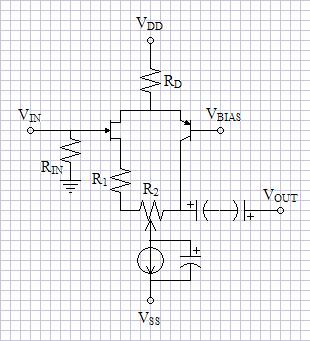This concept is simply implemented with operational amplifiers.  The circuits required for discrete, non-feedback amplifiers might be more complicated than the single-ended purist would like.  However, I submit the circuit of figure 8 as only a suggestion for a possible topology. The folded cascode bends the signal path so that R2 can be in both the numerator and denominator of the gain equation.  The parallel capacitor turns the current source into a automatically-biased voltage.  R1 and R2 will adjust the voltage gain as do the above circuits.  The primary fault of the circuit is that the voltage bias at the wiper of R2 changes with the volume setting, creating a automatic bias readjustment everytime the volume is changed.  Perhaps it is better to use op-amps regardless.

Document History
September 29, 2010  Created